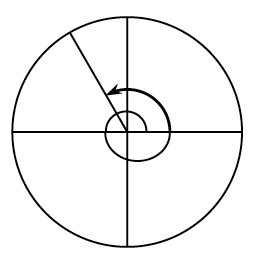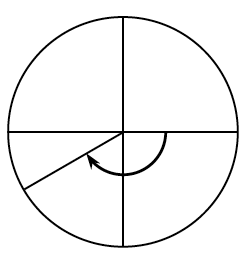### Home > PC3 > Chapter 6 > Lesson 6.1.3 > Problem6-58

6-58.

Below are two of the special angles that are used in the unit circle. Identify the radian measure for each angle shown. Then state the sine, cosine, and tangent of the angle.

1.1.Divide each circle into equal sectors and count them.
Remember that half of a circle is equivalent to $π$ radians.